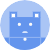search
Search
Guest 0reps
Thanks for the thanks!
close
account_circle
Profile
exit_to_app
Sign out
search
keyboard_voice
close
Searching Tips
Search for a recipe:
"Creating a table in MySQL"
Search for an API documentation: "@append"
Search for code: "!dataframe"
Apply a tag filter: "#python"
Useful Shortcuts
/ to open search panel
Esc to close search panel
to navigate between search results
d to clear all current filters
Enter to expand content previewDoc SearchCode Search BetaSORRY NOTHING FOUND!
mic
Start speaking...Voice search is only supported in Safari and Chrome.
Shrink
Navigate to
A
A
brightness_medium
share
arrow_backShareTwitterFacebook

# NumPy | dot Method

Programming
chevron_right
Python
chevron_right
NumPy
chevron_right
Documentation
schedule Jul 1, 2022
Last updated
local_offer PythonNumPy
Tags

NumPy's dot(~) is an extremely useful method that can be used to compute the product between:

• scalar and scalar

• vector and vector (dot product)

• matrix and vector

• matrix and matrix

# Parameters

1. a | number or array_like

The first argument.

2. b | number or array_like

The second argument.

# Return value

The following table summaries what operation is performed as well as the return type:

a

b

operation

Return type

scalar

scalar

Scalar multiplication

number

1D array

1D array

Vector dot product

number

2D array

1D array

Matrix-vector product

1D Numpy array

2D array

2D array

Matrix-matrix multiplication

2D Numpy array

n-D array

n-D array

Batch products

n-D Numpy array

# Examples

## Dotting two numbers (scalar and scalar)

 np.dot(2, 3) 6 
WARNING

Avoid this for scalar multiplication. If you just want to multiply two scalar numbers, just use the standard 2 * 3 - it's faster and clearer.

## Dotting two arrays (vector and vector)

 # (1 * 3) + (2 * 4) = 11np.dot([1,2], [3,4]) 11 

Mathematically, we're doing the following:

$$\begin{pmatrix} 1\\ 3\\ \end{pmatrix} \cdot \begin{pmatrix} 2\\ 4\\ \end{pmatrix}= 11$$

Just as a side note, the parameters just have to be array-like; we can use NumPy arrays as well:

 x = np.array([1,2])y = np.array([3,4])np.dot(x, y) 11 

## Matrix-vector multiplication

 X = np.array([[1,2],[3,4]])y = np.array([5,6])np.dot(X,y) array([17, 39]) 

Mathematically, we're doing the following:

$$\begin{pmatrix} 1&2\\ 3&4\\ \end{pmatrix} \begin{pmatrix} 5\\ 6\\ \end{pmatrix}= \begin{pmatrix} 17\\ 39\\ \end{pmatrix}$$

## Matrix-matrix multiplication

 x = [[1,0], [0,1]]y = [[2,2], [2,2]]np.dot(x, y) array([[2, 2],       [2, 2]]) 

Mathematically, we're doing the following:

$$\begin{pmatrix} 1&0\\ 0&1\\ \end{pmatrix} \begin{pmatrix} 2&2\\ 2&2\\ \end{pmatrix}= \begin{pmatrix} 2&2\\ 2&2\\ \end{pmatrix}$$

Always remember that parameters just have to be array-like; we can use NumPy arrays as well:

 x = np.array([[1,0], [0,1]])y = np.array([[2,2], [2,2]])np.dot(x, y) array([[2, 2],       [2, 2]]) 
WARNING

Avoid this for matrix multiplication. If you want to take the product of two matrices, use NumPy's matmul(~) method or the @ notation instead.

## Batch products

The dot(~) method can be used to compute multiple products at once, like follows:

 x = [ [[1,0], [0,1]], [[1,1], [1,1]] ]y = [3,4]np.dot(x,y) array([[3, 4],       [7, 7]]) 

In this example, the variable $x$ holds the following two matrices:

$$\begin{pmatrix} 1&0\\ 0&1\\ \end{pmatrix} \;\;\;\; \begin{pmatrix} 1&1\\ 1&1\\ \end{pmatrix}$$

The final line, np.dot(x,y), is performing the following mathematical operations:

$$\begin{pmatrix} 1&0\\ 0&1\\ \end{pmatrix} \begin{pmatrix} 3\\ 4\\ \end{pmatrix}= \begin{pmatrix} 3\\ 4\\ \end{pmatrix}$$
$$\begin{pmatrix} 1&1\\ 1&1\\ \end{pmatrix} \begin{pmatrix} 3\\ 4\\ \end{pmatrix}= \begin{pmatrix} 7\\ 7\\ \end{pmatrix}$$

Note that batch products also for vector-vector product and matrix-matrix product as well.

mail